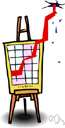# maximize

(redirected from maximizers)
Also found in: Thesaurus, Financial, Encyclopedia.

## max·i·mize

(măk′sə-mīz′)
tr.v. max·i·mized, max·i·miz·ing, max·i·miz·es
1. To increase or make as great or large as possible: "the ideal of maximizing opportunity through the equalizing of educational opportunity" (Robert J. Havighurst).
2. Mathematics To find the largest value of (a function).

max′i·mi·za′tion (-mĭ-zā′shən) n.
max′i·miz′er n.

(ˈmæksɪˌmaɪz) or

## maximise

vb
1. (tr) to make as high or great as possible; increase to a maximum
2. (Mathematics) maths to find the maximum of (a function)
ˌmaximiˈzation, ˌmaximiˈsation, ˌmaxiˈmation n
ˈmaxiˌmizer, ˈmaxiˌmiser n

## max•i•mize

(ˈmæk səˌmaɪz)

v.t. -mized, -miz•ing.
1. to increase to the greatest possible amount or degree: to maximize profits.
2. to give the highest estimate to.
3. to make fullest use of.
[1795–1805]
max`i•mi•za′tion, max`i•ma′tion, n.
max′i•miz`er, n.

## maximize

Past participle: maximized
Gerund: maximizing

Imperative
maximize
maximize
Present
I maximize
you maximize
he/she/it maximizes
we maximize
you maximize
they maximize
Preterite
I maximized
you maximized
he/she/it maximized
we maximized
you maximized
they maximized
Present Continuous
I am maximizing
you are maximizing
he/she/it is maximizing
we are maximizing
you are maximizing
they are maximizing
Present Perfect
I have maximized
you have maximized
he/she/it has maximized
we have maximized
you have maximized
they have maximized
Past Continuous
I was maximizing
you were maximizing
he/she/it was maximizing
we were maximizing
you were maximizing
they were maximizing
Past Perfect
Future
I will maximize
you will maximize
he/she/it will maximize
we will maximize
you will maximize
they will maximize
Future Perfect
I will have maximized
you will have maximized
he/she/it will have maximized
we will have maximized
you will have maximized
they will have maximized
Future Continuous
I will be maximizing
you will be maximizing
he/she/it will be maximizing
we will be maximizing
you will be maximizing
they will be maximizing
Present Perfect Continuous
I have been maximizing
you have been maximizing
he/she/it has been maximizing
we have been maximizing
you have been maximizing
they have been maximizing
Future Perfect Continuous
I will have been maximizing
you will have been maximizing
he/she/it will have been maximizing
we will have been maximizing
you will have been maximizing
they will have been maximizing
Past Perfect Continuous
Conditional
I would maximize
you would maximize
he/she/it would maximize
we would maximize
you would maximize
they would maximize
Past Conditional
I would have maximized
you would have maximized
he/she/it would have maximized
we would have maximized
you would have maximized
they would have maximized
ThesaurusAntonymsRelated WordsSynonymsLegend:
 Verb 1maximize - make as big or large as possible; "Maximize your profits!"maximiseincrease - make bigger or more; "The boss finally increased her salary"; "The university increased the number of students it admitted"minimize, minimise - make small or insignificant; "Let's minimize the risk" 2 maximize - make the most of; "He maximized his role"maximiseexploit, tap - draw from; make good use of; "we must exploit the resources we are given wisely"

## maximize

verb optimize To maximize profit, you need to maximize output.
Translations

## maximize

[ˈmæksɪmaɪz] VT [+ profits, assets, potential, opportunities] → maximizar

## maximize

[ˈmæksɪmaɪz] maximise (British) vt [+ profits, chances, support] →

## maximize

vtmaximieren; (Comput) windowmaximieren, in maximaler Standardgröße darstellen

## maximize

[ˈmæksɪˌmaɪz] vt (profits) → massimizzare; (chances) →

## maximize

vt maximizar
References in periodicals archive ?
"Maximizers are forward thinking, conscientious, optimistic, and satisfied.
In fact, it's often said that optimizers are error maximizers. Here's why: The optimizer works with parameters that are not certain.
The 18 chapters question key tenets of economic models assuming humans to be rational and self-interested maximizers, analyze the structural powers and moral complexities of corporate entities, describe the AIDS treatment policy in Brazil, and advocate for giving cash payments to the poor.
Further research from both economics and psychology [5, 6] suggests the following provoking idea: consumers who exhaustively search for the best product option in the market--maximizers--eventually feel worse than consumers who just look for something "good enough"--called satisficers, despite the fact that maximizers get a better deal.
Firms led by Maximizers for example, seek out risk, believing that no risk is inherently unacceptable.
Chief among them: making good-enough decisions and moving on, a practice known as "sat is-king." (Think "satisfying" plus "sufficing.") Unlike satisficers, my fellow maximizers and I tend to be unhappy with our choices--or so a recent study from Florida State University suggests.
For reasons that are not well understood, in choice situations some individuals ("maximizers") may be driven to identify the best possible outcome, whereas others ("satisficers") may regard any of several possible outcomes as acceptable.
Along the first dimension, club owners may be modeled either as profit maximizers or win maximizers.
But to see families primarily as clusters of self-seeking interest maximizers is to miss what is arguably the most important thing about them: the ongoing and enduring relations of intimacy that bind their members together.
Membership Maximizers will be held throughout the year to help members stay connected and maximize their annual investment in the Chamber.
Now national manager of a job function he innovated, Erdstein leads a team of maximizers as well as hiring and training them.

Site: Follow: Share:
Open / Close